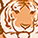Level 1
Level 1# BGT60TR13C Sampling Bandwidth

Hello, I am currently evaluating the BGT60TR13C Radar Sensor with MATLAB mainly for distance measurements. In the Radar SDK online documentation, it is mentioned that the total Bandwidth consists of the sampling Bandwidth + the "gap" from the sampling delay.

Therefore, my impression was that the Bandwidth used to compute the distance corresponds to the sampling Bandwidth (in graph below: from adc_start to adc_end, respectively f_adc_start  to f_adc_end) instead of the total Bandwidth.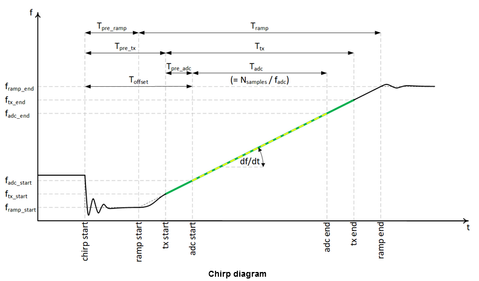But when I compare it with the examples from the SDK (like 'distance_fft.py'), the computed Bandwidth used equals the total Bandwidth:

``````bandwidth_hz = abs(end_frequency_Hz-start_frequency_Hz)
...
self._range_bin_length = (constants.c) / (2 * bandwidth_hz * fft_size /chirpsamples)``````

Question 1: Is the total Bandwidth truly the correct one to utilize for the computation of the range bins and therefore the distance?

Question 2: Is there a way to access the value of the chirp slope S through the c/python/MATLAB functions and which bandwidth is used to compute said slope S (image below for reference)?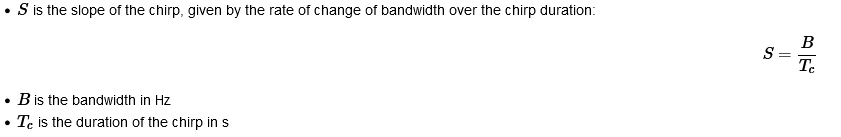Sincerely,
dyang

1 SolutionModeratorModerator# Re: BGT60TR13C Sampling Bandwidth

Hello @dyang ,

Yes, the BGT60TR13 evaluates the chirp across the T_tx interval (T_adc + T_pre_adc) when using the total bandwidth df  (f_tx_end - f_tx_start).

So, the chirp time being computed is the sum of sampling time and sampling delay. See the snippet below, this can be found in DeviceControl.h file.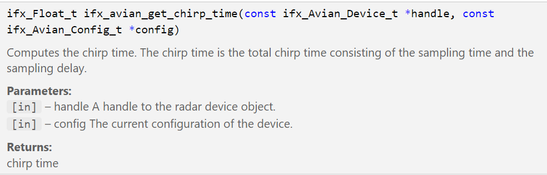Hope this helps, Thankyou

3 RepliesModeratorModerator# Re: BGT60TR13C Sampling Bandwidth

Hey @dyang !

1) Yes, the bandwidth used is the total chirp time (Sampling Bandwidth + sampling delay), you can see the same computed in the code snippet below.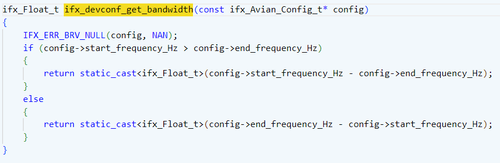2) You can see the slope being used in SpectrumAxis.cpp  which I have shown below (note that IF frequency is also referred to as beat frequency).

Before proceeding, see these these equations.

`IF= 2Sd/c                  here d=1 (as it is computed per meter)IF= S*(2/c)                where S=B/T (B->Bandwidth, T-> Chirp time)IF= (B/T)*(2/c)            (this equation is used in the below show code snippet)`

The same equation is used below,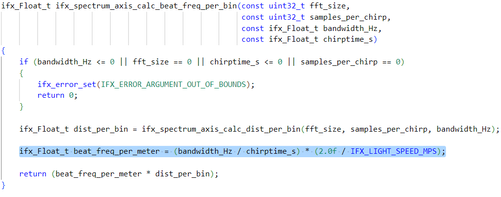Additionally you can also see in the below snippet how T (chirp time) is computed,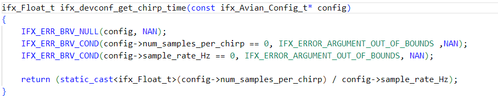I will clarify if the time T computed above is sampling time or sampling time + sampling delay in my next reply.

Thankyou, Siddharth.Level 1
Level 1# Re: BGT60TR13C Sampling Bandwidth

Thank you for the quick reply.
So is it safe to assume that the BGT60TR13 radar "always" evaluates the chirp across the T_tx interval (T_adc + T_pre_adc) when using the total bandwidth df  (f_tx_end - f_tx_start) and given that it is an Avian radar, meaning f_adc_end = f_tx_end, respectively t_adc_end = t_tx_end  according to the Radar SDK Documentation (Image below for reference)?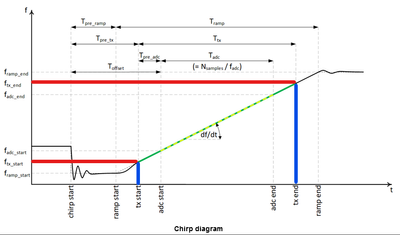Otherwise if it were only the T_adc (without the sampling delay T_pre_adc) interval in conjunction with the total bandwidth df, then the slope S would be steeper than intended resulting in a false computation, no?

I'm looking forward to the post you mentioned about the time T (chirp time) computed.

Sincerely,
dyangModeratorModerator# Re: BGT60TR13C Sampling Bandwidth

Hello @dyang ,

Yes, the BGT60TR13 evaluates the chirp across the T_tx interval (T_adc + T_pre_adc) when using the total bandwidth df  (f_tx_end - f_tx_start).

So, the chirp time being computed is the sum of sampling time and sampling delay. See the snippet below, this can be found in DeviceControl.h file.Hope this helps, Thankyou